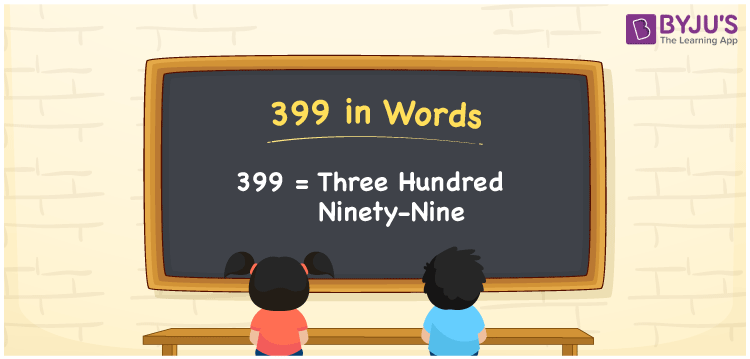# 399 in Words

399 in words is written as Three Hundred Ninety-Nine. For example, if you purchased a badminton shuttlecock worth Rs. 399, then you can say, “I purchased a badminton shuttlecock worth Rupees Three Hundred Ninety-Nine”. 399 is a cardinal number since it shows a certain amount. We usually write numbers in words using ones, tens, hundreds, and so on. Hence, to write number names, a place value chart is very useful. Learn the conversion of number 399 into words in detail in this article.

 399 in Words Three Hundred Ninety-Nine Three Hundred Ninety-Nine in numerical form 399

## 399 in English Words

Generally, we write numbers in words with the help of the English alphabet since English is the commonly used language in the field of education. Therefore, we can write the number 399 in words as Three Hundred Ninety-Nine.## How to Write 399 in Words?

To write numbers in words, it is necessary to find the position of each digit in the given number. The following is the place value chart for the number 399.

 Hundreds Tens Ones 3 9 9

Hence, we can write the expanded form as:

3 x Hundred + 9 x Ten + 9 x One

= 3 x 100 + 9 x 10 + 9 x 1

= 300 + 90 + 9

= 399

= Three Hundred Ninety-Nine

Therefore, 399 in words is written as Three Hundred Ninety-Nine

Interesting way of writing 399 in words

3 = Three

39 = Thirty-Nine

399 = Three Hundred Ninety-Nine

Thus, the word form of the number 399 is Three Hundred Ninety-Nine

399 is a natural number that is one less than 400 and one greater than 398

• 399 in words – Three Hundred Ninety-Nine
• Is 399 an odd number? – Yes
• Is 399 an even number? – No
• Is 399 a perfect square number? – No
• Is 399 a perfect cube number? – No
• Is 399 a prime number? – No
• Is 399 a composite number? – Yes

## Frequently Asked Questions on 399 in Words

### Write 399 in words.

399 in words is Three Hundred Ninety-Nine.

### Simplify 200 + 199, and express in words.

Simplifying 200 + 199, we get 399. Hence, 399 in words is Three Hundred Ninety-Nine.

### What is the place value of 3 in 399?

The place value of 3 in 399 is Hundreds.